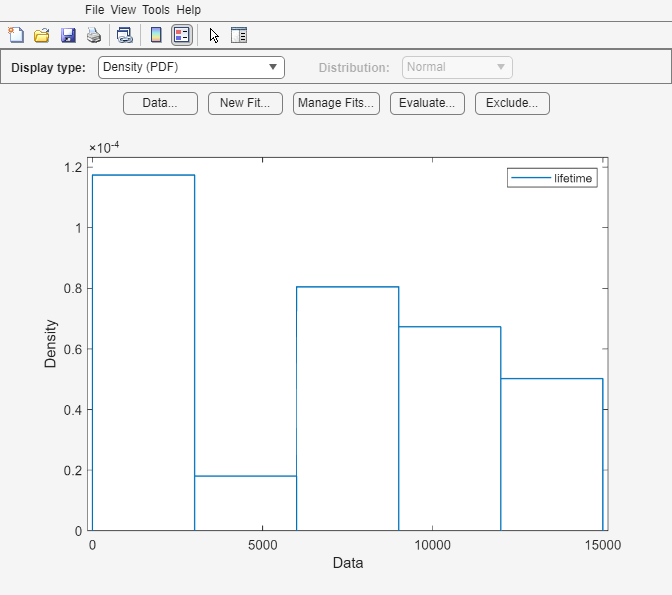# dfittool

Open Distribution Fitter app

The `distributionFitter` function was introduced in R2017a as a replacement for the `dfittool` function. Both functions continue to work to start the Distribution Fitter app.

## Syntax

``dfittool``
``dfittool(y)``
``dfittool(y,cens)``
``dfittool(y,cens,freq)``
``dfittool(y,cens,freq,dsname)``

## Description

This page contains programmatic syntax information for the Distribution Fitter app. For general usage information, see Distribution Fitter.

````dfittool` opens the Distribution Fitter app, or brings focus to the app if it is already open. ```

example

````dfittool(y)` opens the Distribution Fitter app populated with the data specified by the vector `y`.```

example

````dfittool(y,cens)` uses the vector `cens` to specify whether each observation in `y` is censored.```
````dfittool(y,cens,freq)` uses the vector `freq` to specify the frequency of each element of `y`.```
````dfittool(y,cens,freq,dsname)` creates a data set with the name `dsname`, using the data vector, `y`, censoring indicator, `cens`, and frequency vector, `freq`. ```

## Examples

collapse all

Load the `carsmall` sample data.

`load carsmall`

Open the Distribution Fitter app using the `MPG` miles per gallon data.

`distributionFitter(MPG)`The Distribution Fitter app opens, populated with the `MPG` data, and displays the density (PDF) plot. You can use the app to display different plots and fit distributions to this data.

`load lightbulb.mat`

The first column of the data contains the lifetime (in hours) of two types of light bulbs. The second column contains information about the type of light bulb. 1 indicates fluorescent bulbs, and 0 indicates the incandescent bulb. The third column contains censoring information. 1 indicates censored data, and 0 indicates the exact failure time. This is simulated data.

Open the Distribution Fitter app using the first column of `lightbulb` as the input data, and the third column as the censoring data. Name the data `lifetime`.

`distributionFitter(lightbulb(:,1),lightbulb(:,3),[],'lifetime')`To open the Data dialog box, click Data. In the Manage data sets pane, click to highlight the `lifetime` data set row. Finally, to open the View Data Set dialog, click View. The lifetime data appears in the second column and the corresponding censoring indicator appears in the third column.## Input Arguments

collapse all

Input data, specified as an array of scalar values or a variable representing an array of such values.

Data Types: `single` | `double`

Censoring indicator, specified as a vector of 0 and 1 values. The length of `cens` must be equal to the length of `y`. If `y(j)` is censored, then `(cens(j)==1)`. If `y(j)` is not censored, then `(cens(j)==0)`. If `cens` is omitted or empty, then no `y` values are censored.

If you have frequency data (`freq`) but not censoring data (`cens`), then you must specify empty brackets (`[]`) for `cens`.

Data Types: `single` | `double`

Frequency data, specified as a vector of scalar values. The length of `freq` must be equal to the length of `y`. If `freq` is omitted or empty, then all `y` values have a frequency of 1.

If you have frequency data (`freq`) but not censoring data (`cens`), then you must specify empty brackets (`[]`) for `cens`.

Data Types: `single` | `double`

Data set name, specified as a character vector enclosed in single quotes or a string scalar enclosed in double quotes.

If you want to specify a data set name, but do not have censoring data (`cens`) or frequency data (`freq`), then you must specify empty brackets (`[]`) for both `freq` and `cens`.

Example: `'MyData'`

Data Types: `char` | `string`